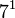# Groups of order 1092

## Contents

This article gives information about, and links to more details on, groups of order 1092
See pages on algebraic structures of order 1092| See pages on groups of a particular order

## Statistics at a glance

The number 1092 has the prime factorization:$\! 1092 = 2^2 \cdot 3^1 \cdot 7^1 \cdot 13^1 = 4 \cdot 3 \cdot 7 \cdot 13$

Quantity Value List/comment
Total number of groups 77
Number of abelian groups (i.e., finite abelian groups) up to isomorphism 2 (number of abelian groups of order$2^2$) times ((number of abelian groups of order$3^1$) times (number of abelian groups of order$7^1$) times (number of abelian groups of order$13^1$) = (number of unordered integer partitions of 2) times (number of unordered integer partitions of 1) times (number of unordered integer partitions of 1) times (number of unordered integer partitions of 1) =$2 \times 1 \times 1 \times 1 = 2$. See classification of finite abelian groups and structure theorem for finitely generated abelian groups.
Number of nilpotent groups (i.e., finite nilpotent groups) up to isomorphism 2 ((number of groups of order 4) times (number of groups of order 3) times (number of groups of order 7) times (number of groups of order 13) =$2 \times 1 \times 1 \times 1 = 2$. See number of nilpotent groups equals product of number of groups of order each maximal prime power divisor, which in turn follows from equivalence of definitions of finite nilpotent group.
Number of supersolvable groups (i.e., finite supersolvable groups) up to isomorphism 71
Number of solvable groups (i.e., finite solvable groups) up to isomorphism 76 See note on non-solvable groups
Number of non-solvable groups up to isomorphism 1 the group projective special linear group:PSL(2,13) (ID: (1092,25))
Number of simple groups up to isomorphism 1 the group projective special linear group:PSL(2,13) (ID: (1092,25))
Number of quasisimple groups up to isomorphism 1 the group projective special linear group:PSL(2,13) (ID: (1092,25))
Number of almost simple groups up to isomorphism 1 the group projective special linear group:PSL(2,13) (ID: (1092,25))
Number of perfect groups up to isomorphism 1 the group projective special linear group:PSL(2,13) (ID: (1092,25))

## GAP implementation

The order 1092 is part of GAP's SmallGroup library. Hence, any group of order 1092 can be constructed using the SmallGroup function by specifying its group ID. Also, IdGroup is available, so the group ID of any group of this order can be queried.

Further, the collection of all groups of order 1092 can be accessed as a list using GAP's AllSmallGroups function.

Here is GAP's summary information about how it stores groups of this order, accessed using GAP's SmallGroupsInformation function:

gap> SmallGroupsInformation(1092);

There are 77 groups of order 1092.
They are sorted by their Frattini factors.
1 has Frattini factor [ 546, 1 ].
2 has Frattini factor [ 546, 2 ].
3 has Frattini factor [ 546, 3 ].
4 has Frattini factor [ 546, 4 ].
5 has Frattini factor [ 546, 5 ].
6 has Frattini factor [ 546, 6 ].
7 has Frattini factor [ 546, 7 ].
8 has Frattini factor [ 546, 8 ].
9 has Frattini factor [ 546, 9 ].
10 has Frattini factor [ 546, 10 ].
11 has Frattini factor [ 546, 11 ].
12 has Frattini factor [ 546, 12 ].
13 has Frattini factor [ 546, 13 ].
14 has Frattini factor [ 546, 14 ].
15 has Frattini factor [ 546, 15 ].
16 has Frattini factor [ 546, 16 ].
17 has Frattini factor [ 546, 17 ].
18 has Frattini factor [ 546, 18 ].
19 has Frattini factor [ 546, 19 ].
20 has Frattini factor [ 546, 20 ].
21 has Frattini factor [ 546, 21 ].
22 has Frattini factor [ 546, 22 ].
23 has Frattini factor [ 546, 23 ].
24 has Frattini factor [ 546, 24 ].
25 - 77 have trivial Frattini subgroup.

For the selection functions the values of the following attributes
are precomputed and stored:
IsAbelian, IsNilpotentGroup, IsSupersolvableGroup, IsSolvableGroup,
LGLength, FrattinifactorSize and FrattinifactorId.

This size belongs to layer 5 of the SmallGroups library.
IdSmallGroup is available for this size.